×#### Thank you for registering.

One of our academic counsellors will contact you within 1 working day.

Click to Chat

1800-1023-196

+91-120-4616500

CART 0

• 0

MY CART (5)

Use Coupon: CART20 and get 20% off on all online Study Material

ITEM
DETAILS
MRP
DISCOUNT
FINAL PRICE
Total Price: Rs.

There are no items in this cart.
Continue Shopping• Complete JEE Main/Advanced Course and Test Series
• OFFERED PRICE: Rs. 15,900
• View Details

```Revision Notes on Quadratic Equations

A polynomial, whose degree is 2, is called a quadratic polynomial. It is in the form of

p(x) = ax2 + bx + c, where a ≠ 0

When we equate the quadratic polynomial to zero then it is called a Quadratic Equation i.e. if

p(x) = 0, then it is known as Quadratic Equation.where a, b, c are the real numbers and a≠0

1. Complete Quadratic Equation  ax2 + bx + c = 0, where a ≠ 0, b ≠ 0, c ≠ 0

2. Pure Quadratic Equation   ax2 = 0, where a ≠ 0, b = 0, c = 0

Let x = α where α is a real number. If α satisfies the Quadratic Equation ax2+ bx + c = 0 such that aα2 + bα + c = 0, then α is the root of the Quadratic Equation.

As quadratic polynomials have degree 2, therefore Quadratic Equations can have two roots. So the zeros of quadratic polynomial p(x) =ax2+bx+c is same as the roots of the Quadratic Equation ax2+ bx + c= 0.

Methods to solve the Quadratic Equations

There are three methods to solve the Quadratic Equations-

1. Factorisation Method

In this method, we factorise the equation into two linear factors and equate each factor to zero to find the roots of the given equation.

Step 1: Given Quadratic Equation in the form of ax2 + bx + c = 0.

Step 2: Split the middle term bx as mx + nx so that the sum of m and n is equal to b and the product of m and n is equal to ac.

Step 3: By factorization we get the two linear factors (x + p) and (x + q)

ax2 + bx + c = 0 = (x + p) (x + q) = 0

Step 4: Now we have to equate each factor to zero to find the value of x.These values of x are the two roots of the given Quadratic Equation.

2. Completing the square method

In this method, we convert the equation in the square form (x + a)2 - b2 = 0 to find the roots.

Step1: Given Quadratic Equation in the standard form ax2 + bx + c = 0.

Step 2: Divide both sides by a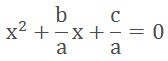Step 3: Transfer the constant on RHS then add square of the half of the coefficient of x i.e.on both sides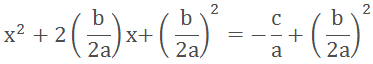Step 4: Now write LHS as perfect square and simplify the RHS.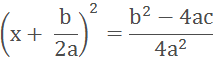Step 5: Take the square root on both the sides.Step 6: Now shift all the constant terms to the RHS and we can calculate the value of x as there is no variable at the RHS.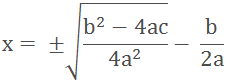In this method, we can find the roots by using quadratic formula. The quadratic formula iswhere a, b and c are the real numbers and b2 – 4ac is called discriminant.

To find the roots of the equation, put the value of a, b and c in the quadratic formula.

Nature of Roots

From the quadratic formula, we can see that the two roots of the Quadratic Equation are -Where D = b2 – 4ac

The nature of the roots of the equation depends upon the value of D, so it is called the discriminant.∆ = Discriminant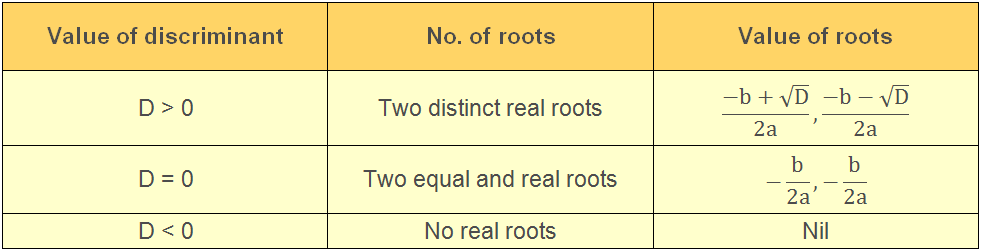```### Course Features

• 728 Video Lectures
• Revision Notes
• Previous Year Papers
• Mind Map
• Study Planner
• NCERT Solutions
• Discussion Forum
• Test paper with Video Solution